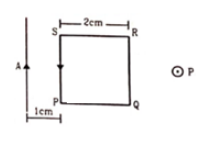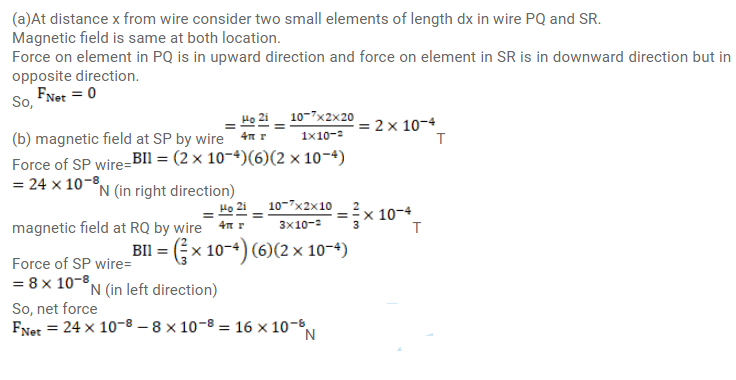# A square loop PQRS carrying a current of 6.0A

Question:

A square loop PQRS carrying a current of $6.0 \mathrm{~A}$ is placed near a long wire carrying $10 \mathrm{~A}$ as shown in figure. (a) Show that the magnetic force acting on the part $P Q$ is equal and opposite to that on the part Rs. (b) Find the magnetic force on the square loop.Solution: